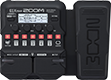# robs cleaj preset

Discussion in 'Zoom G1/G1X Four' started by Rob Mann, Sep 4, 2023.

1. robs cleaj presetDevice: Zoom G1X Four
Firmware: 2.00

Name on device: Robsclean
Optimized for: Phones/Speaker

Effects chain:super chorus, soft delay

Effect: "SuperCho" (Modulation), active - "yes"
"Depth" = 50
"Rate" = 50
"Tone" = 85
"Mix" = 42

Effect: "StereoCho" (Modulation), active - "yes"
"Depth" = 80
"Rate" = 30
"Tone" = 0
"Mix" = 60

Effect: "Gt GEQ 7" (Filter), active - "yes"
"100" = 2.5
"200" = -1.5
"400" = -4.0
"800" = -3.0
"1.6k" = 0.0
"3.2k" = 1.0
"6.4k" = -1.0
"VOL" = 100

Effect: "AnalogDly" (Delay), active - "yes"
"Time" = 429
"F.B." = 14
"Mix" = 51
"Tail" = On

Effect: "BlackOpt" (Dynamics), active - "yes"
"Comp" = 50
"Lo" = 50
"Hi" = 50
"Volume" = 100

Patch Volume: 120